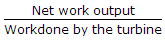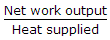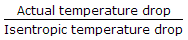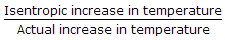# Mechanical Engineering - Thermodynamics

Exercise : Thermodynamics - Section 4
46.
An open system is one in which
heat and work crosses the boundary of the system, but the mass of the working substance does not crosses the boundary of the system
mass of the working substance crosses the boundary of the system but the heat and work does not crosses the boundary of the system
both the heat and work as well as mass of the working substance crosses the boundary of the system
neither the heat and work nor the mass of the working substance crosses the boundary of the system
Explanation:
No answer description is available. Let's discuss.

47.
One reversible heat engine operates between 1600 K and T2 K and another reversible heat engine operates between T2 K and 400 K. If both the engines have the same heat input and output, then temperature T2 is equal to
800K
1000K
1200K
1400K
Explanation:
No answer description is available. Let's discuss.

48.
When the gas is heated at constant volume, the heat supplied
increases the internal energy of the gas and increases the temperature of the gas
does some external work during expansion
Both (A) and (B)
None of these
Explanation:
No answer description is available. Let's discuss.

49.
The efficiency of a gas turbine is given by